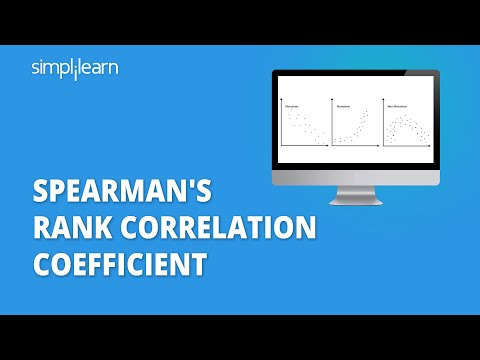### Tutorial PlaylistCorrelation is a statistical measure that determines how closely two variables fluctuate.  A positive correlation shows the extent to which those variables increase or decrease in parallel. A negative correlation shows the range in which one variable increases as the other decreases. In this article, we will discuss one such correlation i.e Spearman’s Rank Correlation.

#### Post Graduate Program in Data Analytics

In partnership with Purdue University## What Is Monotonic Function?

To understand Spearman’s rank correlation, it is important to understand monotonic function. A monotonic function is one that either never increases or never decreases as its independent variable changes.

The following graph illustrates the monotonic function:• Monotonically Increasing: As the variable X increases, the variable Y never decreases.
• Monotonically Decreasing: As the variable X increases, the variable Y never increases.
• Not Monotonic: As the X variable increases, the Y variable sometimes decreases and sometimes increases.

## Spearman’s Rank Correlation

Spearman’s rank correlation measures the strength and direction of association between two ranked variables. It basically gives the measure of monotonicity of the relation between two variables i.e. how well the relationship between two variables could be represented using a monotonic function.

The formula for Spearman’s rank coefficient is:𝝆 = Spearman’s rank correlation coefficient

di = Difference between the two ranks of each observation

n = Number of observations

The Spearman Rank Correlation can take a value from +1 to -1 where,

• A value of +1 means a perfect association of rank
• A value of 0 means that there is no association between ranks
• A value of -1 means a perfect negative association of rank

Let’s understand the concept better with the help of an example.

#### FREE Course: Introduction to Data Analytics

Learn Data Analytics Concepts, Tools & Skills## Example of Spearman’s Rank Correlation

Consider the score of 5 students in Maths and Science that are mentioned in the table.Step 1: Create a table for the given data.

Step 2: Rank both the data in descending order. The highest marks will get a rank of 1 and the lowest marks will get a rank of 5.

Step 3: Calculate the difference between the ranks (d) and the square value of d.Step 5: Insert these values into the formula.= 1 - (6 * 14) / 5(25 - 1)

= 0.3

The Spearman’s Rank Correlation for the given data is 0.3. The value is near 0, which means that there is a weak correlation between the two ranks.

Get broad exposure to key technologies and skills used in data analytics and data science, including statistics with the PG Program in Data Analytics.

## Conclusion

In this tutorial ‘The Definitive Guide To Understand Spearman’s Rank Correlation’, you saw the concept of Spearman’s Rank Correlation and how to find its rank coefficient. You also worked on an example to understand how you can find the association between the ranks of the given data.

If you are looking to pursue a career as a Data Analyst, Simplilearn’s Data Analytics PGP in partnership with Purdue University & in collaboration with IBM is the program for you.

Was this tutorial on Spearman’s Rank Correlation useful to you? Do you have any doubts or questions for us? Mention them in this article's comments section, and we'll have our experts answer them for you at the earliest!Aryan Gupta